### Calculate foreign exchange rates with this free currency converter!

Note: This service will give you a quick idea of currency values. Please consult a bank if you need a more precise conversion. AsiaOne takes no responsibility for any errors in the rates used in this service.

#Good time to change /Lower than 6 months average

•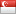SGD 1 = 1.132631 AUD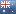AUD 1 = SGD 0.8829
2 hours ago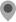Check location

•SGD 1 = 0.978473 CAD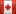2 hours agoCheck location

•SGD 1 = 0.660501 CHF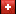CHF 1 = SGD 1.514
3 hours agoCheck location

•SGD 1 = 5.320000 CNY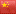CNY 100 = SGD 18.796992
2 hours agoCheck location

•SGD 1 = 0.685400 EUR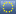EUR 1 = SGD 1.459
3 hours agoCheck location

•SGD 1 = 0.595770 GBP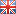GBP 1 = SGD 1.6785
3 hours agoCheck location

•SGD 1 = 5.704500 HKD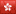HKD 100 = SGD 17.53002
2 hours agoCheck location

•SGD 1 = 11210.000000 IDR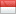IDR 1000 = SGD 0.089206
3 hours agoCheck location

•SGD 1 = 63.000000 INR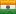INR 100 = SGD 1.587301
3 hours agoCheck location

•SGD 1 = 107.642626 JPY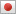JPY 1000 = SGD 9.29
2 hours agoCheck location

•SGD 1 = 964.321100 KRW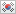KRW 1000 = SGD 1.036998
2 hours agoCheck location

•SGD 1 = 3.422000 MYR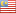MYR 100 = SGD 29.222676
3 hours agoCheck location

•SGD 1 = 1.220000 NZD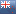NZD 1 = SGD 0.819672
2 hours agoCheck location

•SGD 1 = 41.601000 PHP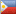PHP 100 = SGD 2.403788
2 hours agoCheck location

•SGD 1 = 26.220000 THB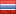THB 100 = SGD 3.813882
5 hours agoCheck location

•SGD 1 = 23.370000 TWD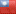TWD 100 = SGD 4.27899
3 hours agoCheck location

•SGD 1 = 0.731528 USD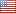USD 1 = SGD 1.367
2 hours agoCheck location

•SGD 1 = 17670.000000 VND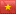VND 100000 = SGD 5.659309
3 hours agoCheck location